# Loss and Impedance Measurements of Reactors in Operation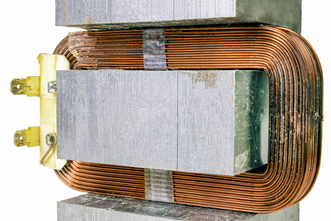Introduction

A reactor is a component that uses induction coils to suppress inrush current and harmonics. In the power system industry and the power electronics industry such as inverters and converters, it is called a reactor, and in the electronic components industry such as electronic circuits, it is called an inductor (Table 1).

In recent years, there has been a growing need to improve the performance of power conditioning systems for photovoltaic and wind power generation and inverters for EVs. Reactors used in their boost converters are important components.

This document covers the reactors in the power electronics industry.

 Table 1  Comparison of reactors and inductors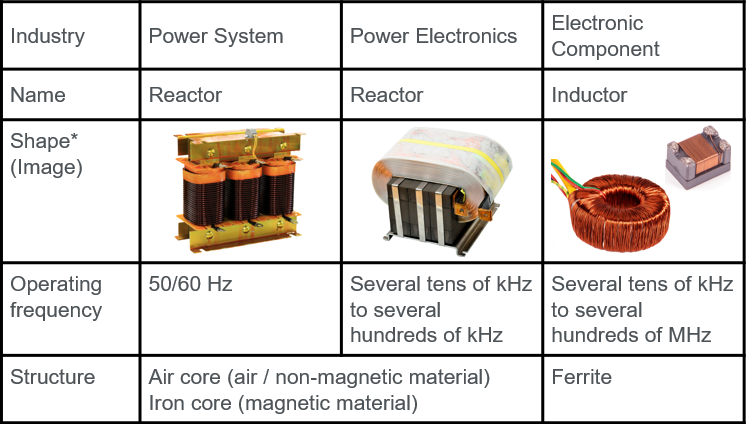* The images are for illustration purposes only. Actual products vary in shape and specifications.

Types and applications of reactors

Reactors are classified by structure, connection method, and purpose of use, but they are electrically classified into two types: AC reactors and DC reactors.

AC reactor

Purpose: Protection against inrush current, harmonic suppression and power factor improvement

An AC reactor is inserted in the input or output side of a power  converter such as an inverter. Insertion of an AC reactor in the input side is effective in protecting against inrush current into the inverter and insertion in the output side suppresses distortion and harmonics contained in AC signals.

Suppressing the harmonics of currents can improve power factor. It is also effective in suppressing surge voltage.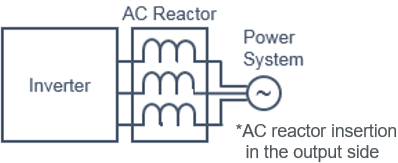DC reactor

Purpose: Smoothing currents and suppressing harmonics

A DC reactor can smooth pulsed or pulsating currents flowing in a DC circuit and suppress the harmonic components of the currents.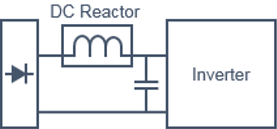In a step-up/step-down chopper circuit, an input DC voltage is  stepped up or stepped down according to the ON/OFF time ratio. The key component of the circuit is the DC reactor. A current flows when the chopper circuit is ON/OFF, and the DC reactor provides a feedback effect of making the output voltage constant based on the time ratio.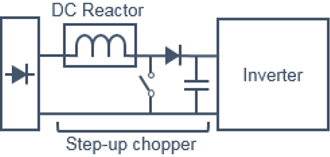Understanding reactor characteristics

For ideal reactors (or inductors), the reactance is called  inductive reactance and expressed as XL. The inductive reactance is defined as XL = 2πfL and causes the current phase to lag by 90°.

 E (Voltage) [V] f (Frequency) [Hz] I (Current) [A] L (Inductance) [H] XL (Reactance) = 2πfL [Ω] Z (Impedance) = -jXL = -j(2πfL) [Ω]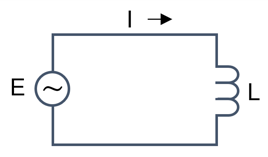In practice, a reactor has a resistance component and parasitic capacitance. The effects of environment, including changes in temperature, also need to be evaluated. For that, it is essential to accurately capture the characteristics by using measuring instruments.

Challenges / Demands

Measurement of high-frequency drive reactor in operation

LCR meters, impedance analyzers, and network analyzers are  commonly used to measure resistance, capacitance, inductance, impedance, and reactance of electronic components (Table 2). As these instruments apply a sine wave of internal signal source to the electronic components to measure the characteristics, they cannot measure the loss and harmonics in an actual operation state. In addition, if there is a DC component in the input signal, the input channel of the measuring instrument may be saturated or damaged.

For a reactor of iron core structure, it is necessary to measure the changes in magnetic flux density, impedance, and reactance according to the input signal level under actual operating conditions. Although depending on the frequency band, power meters/power analyzers are suitable for measuring reactors in operation.

Items to be measured in an operation state include:

lLoss
lImpedance, reactance, inductance and resistance
lHarmonic (13th and higher) suppression effect

Need for wide bandwidth and high-speed sampling

A square wave used for switching includes higher harmonics as well as DC components. The level of the nth harmonic is 1/n, and only after the 11th harmonic it goes below -20 dB (1/10). For this reason, a square wave measurement requires a measuring instrument with a bandwidth of DC to a frequency 10-20 times of the input signal frequency. For instance, a bandwidth of 1-2 MHz or higher is required for a system operating at a switching frequency of 100 kHz. In particular, the rising and falling edges of a square wave are steep. To accurately measure power values, the square waveform must be accurately converted to digital values, which requires a wide bandwidth and higher speed sampling.

On the other hand, if the instrument’s measurement bandwidth is too wide for the measurement target bandwidth, unwanted noise may affect the measurement. Depending on the situation, filters or averaging functions should be used appropriately.

Measurement of high voltage and large current

As reactors in the fields of power systems and power electronics are high in voltage and large in current, a power analyzer, probe and current sensor with a bandwidth and input range that can accept input of the voltage and current to be measured, and connections and wirings that eliminate noise and measurement errors are required. Especially for measuring a reactor driven at high frequency, a sensor capable of measuring from DC is needed because DC components are superimposed.

Measurement under low power factor conditions

The phase difference between voltage and current of a reactor is approximately 90 ° and the power factor is close to zero. In measurements using a power analyzer, a small phase error during measurement greatly affects the results. It is essential  to select a power analyzer with a function to correct phase difference including a current sensor and specifications that enable high-precision measurements even at a low power factor.

Table 2  Comparison of instruments that can measure reactors

 Power Analyzer LCR Meter Impedance Analyzer Network Analyzer Original measurement item Power parameters LCR value AC impedance S parameters Purpose Power parameters, efficiency of power system, invertor etc. Characteristics of electronic components Characteristics of high impedance electronic components or materials of 10 kΩ or more Characteristics of low impedance electronic components or materials of 10 kΩ or less Bandwidth up to 1 MHz (Power analyzer) up to 20 MHz (PX8000) up to a few MHz up to a few GHz up to 100 GHz Measuring method Calculate from voltage waveform and current waveform in actual operation Measure and calculate by applying a sine wave of a specific frequency using an equivalent circuit model By changing the frequency of  input signal, DC and AC components are obtained and combined. Sweep the frequency of input signal to measure and calculate reference / reflection / transmission signals Display Power parameter values and trend waveforms Input voltage / current waveform Numerical values of LCR, etc. Graph display of voltage and current with respect to parameter frequency Smith chart, logarithmic amplitude, phase, group delay, etc. Advantages Measurement in an operation state Harmonic analysis Easy and high-speed measurement just by connecting Low price Measurement in a wide frequency band Equivalent circuit analysis function Resonance analysis Measurement in GHz band Equivalent circuit analysis function Disadvantages Cannot measure in high frequency bands Knowledge of equivalent models required Frequency characteristics cannot be measured. Measured values change  depending on the connection with electronic components. Be careful with probes and jigs. Cannot measure low frequency bands Expensive

Proposal / Solution

YOKOGAWA’s Precision Power Scope PX8000 and Precision Power Analyzer WT5000 enable measurement of reactor’s loss and impedance in operation.

Measurement bandwidth of power analyzer

The WT5000 with a 1 MHz measurement bandwidth is sufficient for measurements of reactors for 50/60 Hz power supply systems, but we recommend using the PX8000 with a 20 MHz bandwidth for more accurate measurements of reactors used in applications with high switching frequencies, such as power conditioning systems and EV batteries.

Observation of voltage and current waveforms

The WT5000 can sample voltage and current waveforms at 10 MS/s and the PX8000 can at 100 MS/s respectively to display them. There is no need to connect an oscilloscope for waveform observation. Since high voltage and high frequency voltage are measured, it may be necessary to use a damping resistor in some cases.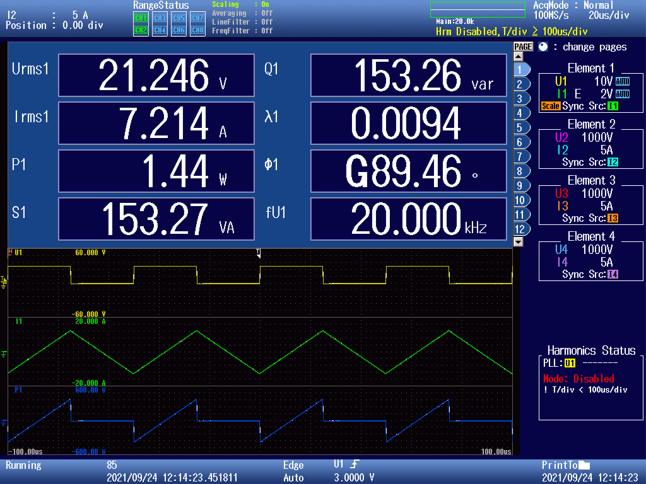Example of simultaneous measurement and display of power parameters and waveforms on PX8000  (A function generator is used for the input.)

Measurement of reactor loss

One of the factors that reduce the efficiency of an entire system is the reactor, and the first step in improving the efficiency is to accurately grasp the degree of reactor loss. In an actual operation state, a skin effect due to high current and high frequency and changes in the properties of magnetic materials due to temperature rise are also expected to affect the efficiency.

Method 1

Reactor’s power consumption P (total loss) is directly measured from the current flowing in the reactor and the voltage between the reactor’s both ends. The phase difference between the applied voltage and the flowing current is 90°, which means that the power factor is very low. However, measurement errors of the WT5000 and the PX8000 at zero power factor are very small, which allows high-precision measurements.

In this method, the common mode voltage is directly applied to the power analyzer, making it easy for noise to enter. Additionally, since the system under measurement is driven by a high-voltage switching waveform (square wave), anti-noise measures are essential for the entire measurement system.

Iron loss can be obtained by subtracting the copper loss calculated from the reactor winding resistance r and the current value I from the total loss P.

P(iron loss) = P(total loss) - r(winding resistance) x I(current)^2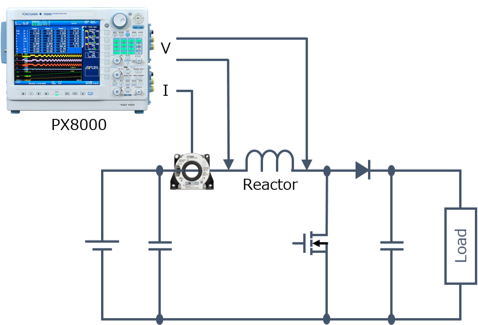In iron loss measurement of magnetic materials, the loss can also be obtained by measuring the B-H hysteresis loop. When the vertical axis is magnetic flux density B and the horizontal axis is magnetic field strength H, the area inside the hysteresis loop represents the iron loss. The PX8000 also supports this calculation.

Method 2

This method measures power from the voltage and current before and after the reactor and then calculates the reactor loss.

Reactor Loss = Pin - Pout

In this method, the effect of noise due to common-mode voltage can be reduced.

However, the deviation between input channels (elements) results in a measurement error. This error can be reduced by taking two measurements with the input channels swapped  and averaging the results.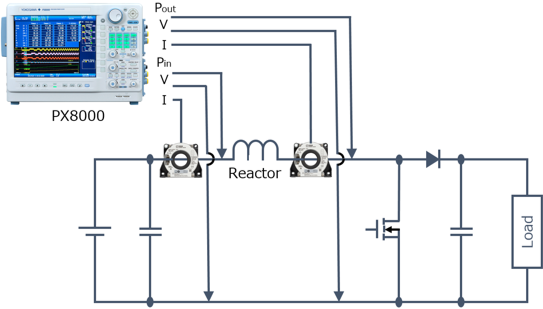Measurement of reactor impedance

Apply AC signal E (frequency f) to the reactor, and measure the reactor loss, voltage U, current I, and phase difference θ  with a power analyzer. Impedance, reactance, inductance, and resistance components can be calculated using a user-defined  computation function.

 Impedance    Z = U / I Reactance     X = Z x sin (θU − θI) Inductance     L = X / 2πf Resistance     R = Z x cos (θU − θI)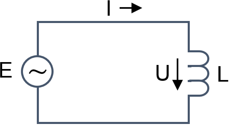Alternatively, you can refer to parameter calculation such as impedance reluctance for each order of the switching frequency of a series/parallel equivalent circuit, which is calculated using the harmonic measurement function. For details, see page “App-16" of the PX8000 Precision Power Scope Features Guide.

Noise countermeasures

To protect against noise is especially important measurements under low power factor conditions.

lAs far as possible, measure on the ground side circuit.
lKeep the measurement target, wiring cables, and power analyzer away from the equipment generating noise.
lKeep the wiring cables as short as possible. Make them into a twisted pair.
lWrap the cables with an electrostatic shielding cover and connect them to ground.
lCover the equipment with a metal cover.

For accurate measurements

A current sensor has a through type structure, and the current flowing through the primary wiring is detected by the winding of the electromagnetic core of the current sensor. This makes it necessary to pay attention to the following points.

1.Choose a current sensor with appropriate rated current and frequency bandwidth. Also pay attention to the current range setting on the power analyzer.
2.Wiring Considerations
lPlace the primary wiring in the center of the current sensor.
lMake sure that the primary wiring and secondary wiring do not interfere with each other. Make the secondary wiring as short as possible and maintain its distance from the primary wiring, without allowing them to be parallel to each other.
lAWG24 or higher is recommended for the secondary wiring material. A twisted pair cable may be more suitable than a shielded cable for inverter measurements.
lSecure reproducibility by fixing the current sensor and cables.
3.Use the amplitude/phase correction function.

Since the measurement of a reactor is performed under a condition that the power factor is close to zero, a slight phase shift results in a measurement error. When an AC/DC current sensor or current clamp probe is used, its  amplitude error and the phase difference between the voltage and current signals can be corrected to enable more accurate power measurement.

The PX8000 and WT5000 have a phase difference correction (de-skew) function. In the case of reactor measurement using a current sensor, this function corrects the phase difference (transmission time difference) of the current sensor and reduces the error factor on the time axis  at the drive frequency of the reactor.

4.Use individual Null to remove offsets.

Null is a function that resets the offset value to zero while wired, including an external current sensor. This function is especially effective for step-up/step-down converters and chopper circuits, where DC components are superimposed. Null can be individually set to ON, HOLD, or OFF for each input. It is recommended that you perform zero level compensation (a function to compensate for the zero level in the internal circuit) before executing the null function. Be sure to warm up the instrument before you perform null or zero level correction.

Improvement of reproducibility by correcting an entire measurement system

To improve reproducibility, use a function generator and a high frequency amplifier to apply the highest possible current to a standard capacitor (or inductor) whose loss (W) is known at the frequency at which the standard capacitor is actually switched and driven, and perform correction by adjusting the time difference data while measuring the capacitor loss with a WT5000 or PX8000. Then, the measured capacitor loss is adjusted to be closer to the pre-specified loss of standard capacitor with a correction function.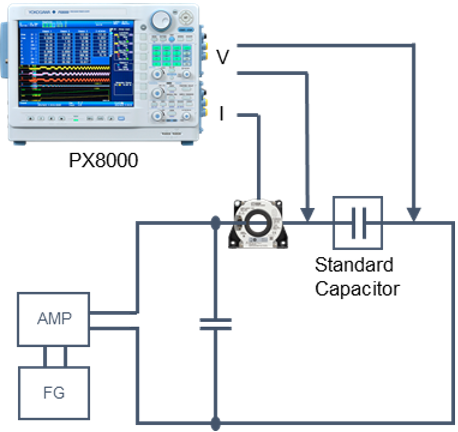Conclusion

lThe PX8000 or WT5000 is ideal for measuring reactors in an operation state.
lWiring and placement need to be made so as to eliminate noise.
lBy utilizing the phase difference correction (de-skew) function of the power analyzer, measurement error can be reduced at the driving frequency of a reactor.
lCorrection values can be obtained for an entire measurement system including a current sensor and wiring by using a standard capacitor.

### Related Products & Solutions

#### PX8000 - Precision Power Scope

• Transient/Start-up Waveform Capture & Power Analysis
• Harmonics, Cycle by cycle and FFT analysis

#### WT5000 - Precision Power Analyzer

• Yokogawa WT5000 precision power analyzer with superior accuracy
• 7 swappable input elements
• 4 motor inputs
• Harmonics test compliant to IEC 61000-3-2

#### Power Analyzers and Power Meters

Yokogawa, the world's largest manufacturer of energy and power analyzers and meters, provides a broad choice of digital power analyzers to satisfy all requirements.

Top